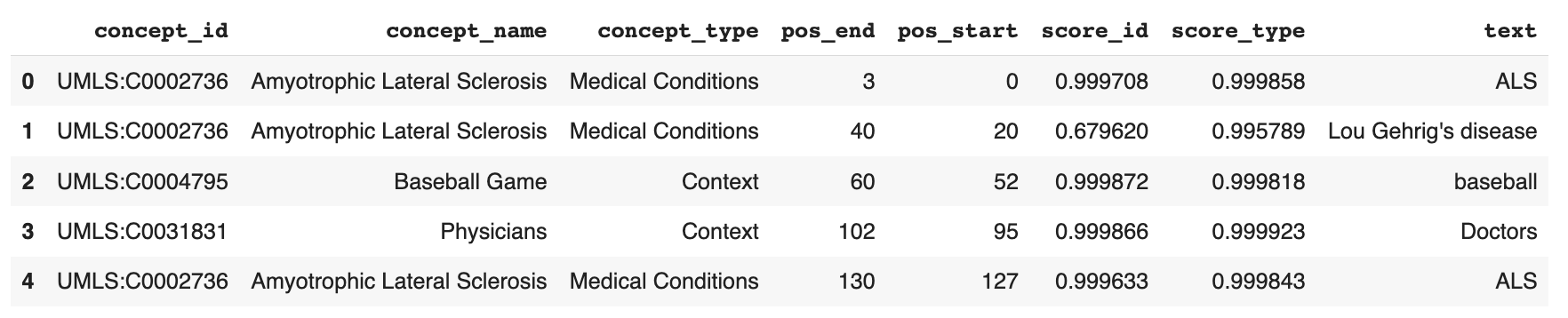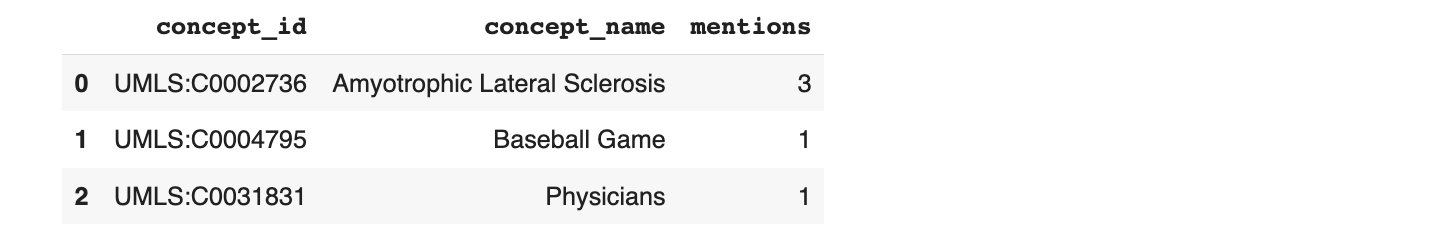# Analytics with Pandas

ScienceIO is better with pandas.

Pandas allows you to create a DataFrame that enables easier viewing and exploration of results, such as when you perform analytical operations like filtering.

In this example, we'll make a request to the API's `structure` endpoint, pass the results directly to a pandas DataFrame, and display the results in a table using the pandas json_normalize operation.

``````# Import the ScienceIO library
from scienceio import ScienceIO

# Create a scio object
scio = ScienceIO()

# Submit the request
query_text = "ALS is often called Lou Gehrig's disease, after the baseball player who was diagnosed with it. Doctors usually do not know why ALS occurs."

response = scio.structure(query_text)

# Use pandas to display the results in a table
import pandas as pd
df = pd.json_normalize(response['spans'])
df
``````

The results display like this:Now, you could perform further analysis by using pandas to get a count of how often each concept was mentioned. Do this with the pandas groupby operation, and aggregate by `concept_id` and `concept_name`:

``````# Aggregate by concept_id and concept_name
df.groupby(["concept_id", "concept_name"]).count()["pos_start"] \
.reset_index() \
.sort_values(by="pos_start", ascending=False) \
.rename(columns={"pos_start": "mentions"}) \
.reset_index(drop=True)
``````

The results look like this: